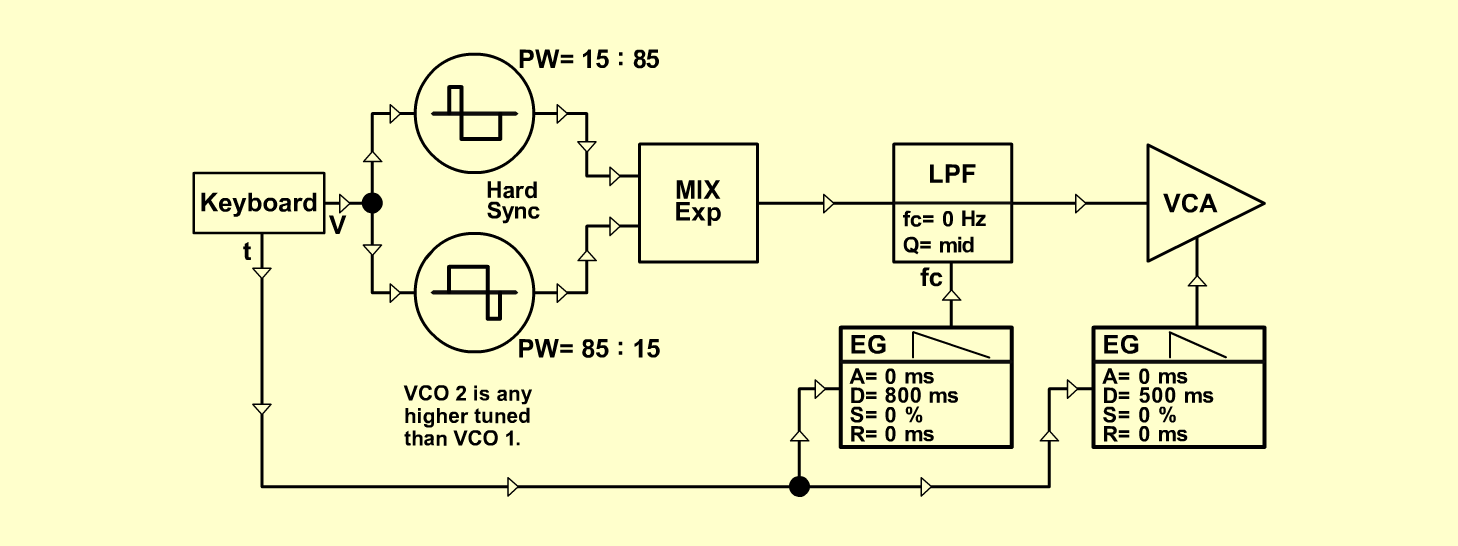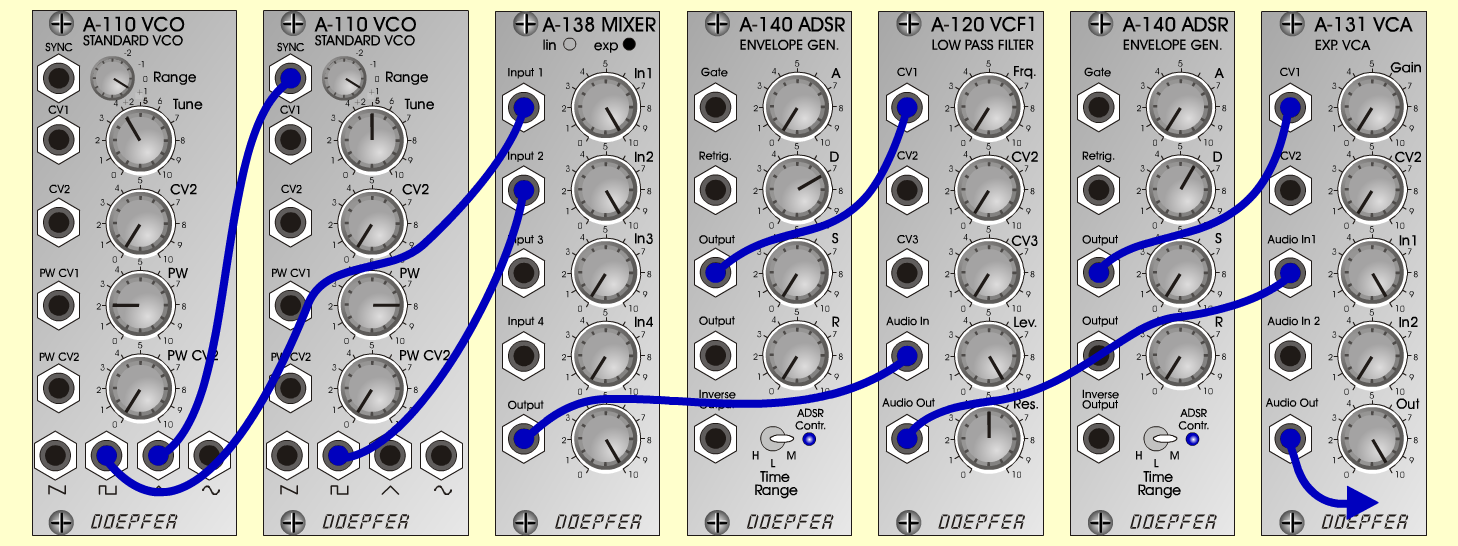PizzicatoInstrumentsD o e p f e r A - 100 Connections: Settings: A-110/1 (Pulse) <=>  A-138/exp (Input 1)   A-110/1 (Triangle) <=>  A-110/2 (Sync)   A-110/2 (Pulse) <=>  A-138/exp (Input 2)   A-138/exp (Out) <=>  A-120 (Audio In)   A-140/1 (Output) <=>  A-120 (CV 1)   A-120 (Audio Out) <=>  A-131 (Audio In)   A-140/2 (Output) <=>   A-131 (CV 1)   A-131 (Out) <=> Amplifier   Pitch: KB or Seq   Trigger and Gate: KB, Seq or   A-145 (Square) <=>  A-180   A-180 <=>  A-140/1+2 (Gate) A-110/1 (Range = +1, Tune = 4, PW = 2)   A-110/2 (Range = +1, Tune = 5, PW = 8)   A-138/exp (In 1 = 10, In 2 = 10, Out = 10)   A-120 (Freq = 0, Lev = 10, Res = 5)   A-140/1 (A = 0, D = 7, S = 0, R = 0, Range = M)   A-140/2 (A = 0, D = 6, S = 0, R = 0, Range = M)   A-131 (Gain = 0, Audio In 1 = 10, Audio Out = 10)   A-145 (Frq = 1, Range = M) Notes: Tune A-110/2 any higher than A-110/1.   Change the sound: connect the    A-140/1-Output  <=> A-120 (CV 2)    and set the A-120 (CV 2) lower as 6. Josef MuellerSound samples Whistle Siciliano (Bach BWV 1031)     Melody - Instrument: 'Whistle' Pizzicato (261 Hz) Pizzicato (523 Hz)